Open in App
Not now

# Class 8 RD Sharma Solutions – Chapter 15 Understanding Shapes Polygons – Exercise 15.1

• Last Updated : 18 May, 2021

### Question 1. Draw a Rough diagram to illustrate the Following.

(i) Open curve

(ii) Closed curve

Solution:

i) Open curve: A curve in which the beginning point and the ending point do not meet each other is known as an open curve.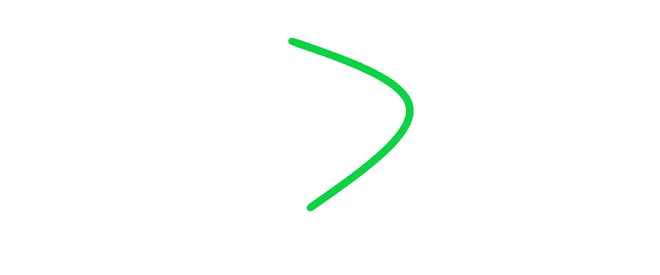ii) Closed curve: A curve in which the beginning point and endpoint meet at each other or cuts each other is known as a closed curve.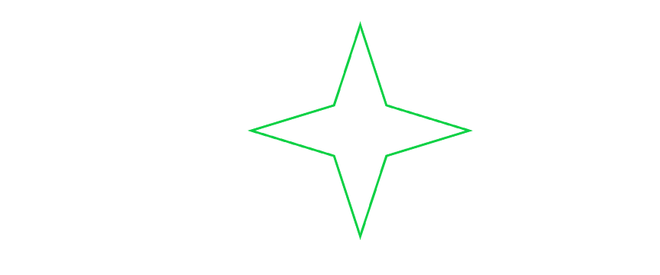### Question 2. Classify the following as open or closed.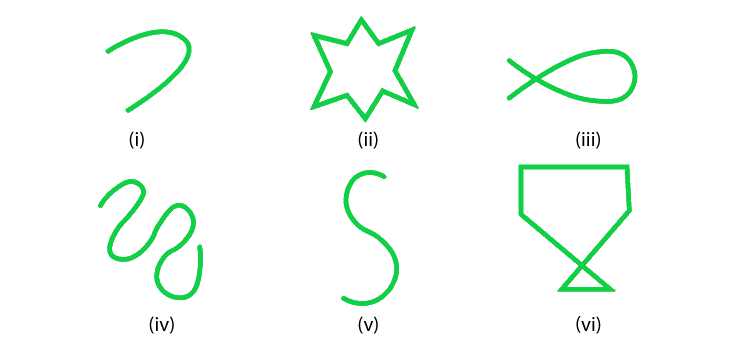Solution:

• open curve:  A curve in which starting point and ending point are different or do not cut each other .
• closed curve: A curve in which starting point and ending point are same and cut each other.

Using the above definition we can classify the given figures as follows :

i) open curve (as both stating and ending points are different)

ii) closed curve (as both the points are same )

iii) closed curve (as both the points cut each other)

iv) open curve (as both starting point and ending point are different)

v) open curve (as both starting point and ending point are different)

vi) closed curve (as both starting point and ending point meet at same point)

### Question 3. Draw a polygon and shade it’s interior. Also draw its diagonals, if any:

Solution:

In polygon ABCD, AC and BD are the diagonals of a polygon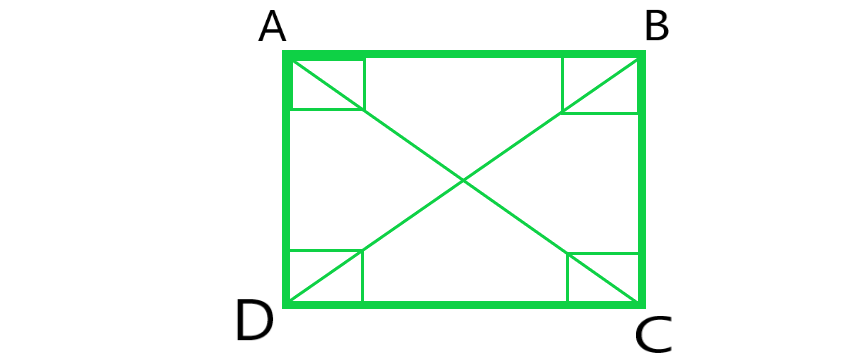### Question 4. Illustrate if possible, each one of the following with a rough diagram.

(i) A closed curve that is not a polygon.

(ii) An open curve made up entirely of line segments.

(iii) A polygon with two sides.

Solution:

(i) A closed curve that is not a polygon.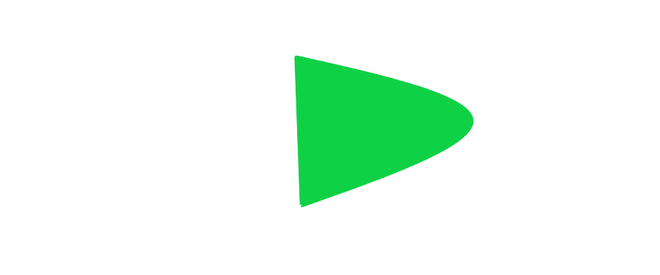(ii) An open curve made up entirely of line segments.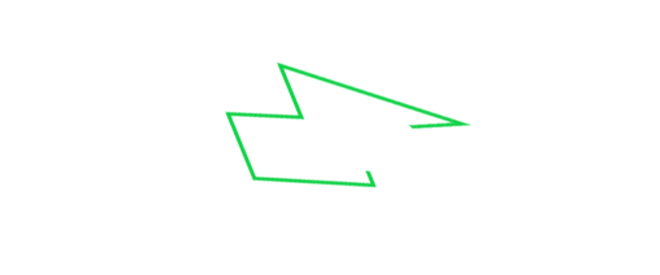(iii) A polygon with two sides.

A polygon with two sides is not possible because, a polygon should have minimum three sides.

### Question 5. Following are some figures: Classify each of these figures on the basis of the following:

(i) Simple curve

(ii) Simple closed curve

(iii) Polygon

(iv) Convex polygon

(v) Concave polygon

(vi) Not a curve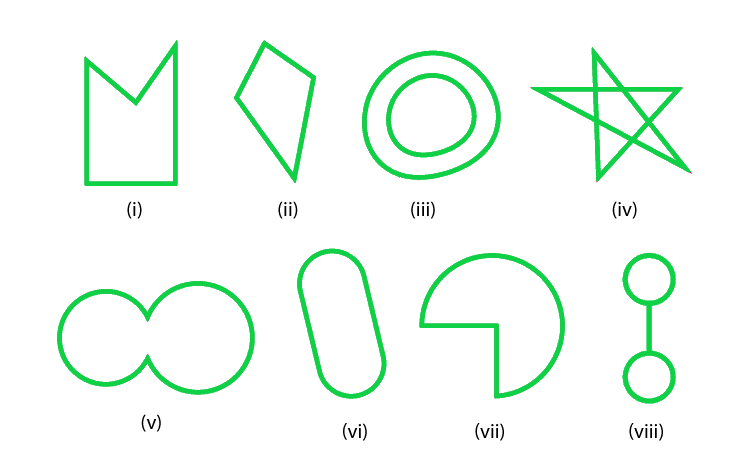Solution:

(i) It is a Simple Closed curve and a concave polygon. This is a simple closed curve and as a concave polygon all the vertices are not pointing outwards.

(ii) It is a Simple closed curve and a convex polygon. This is a simple closed curve and as a convex polygon all the vertices are pointing outwards.

(iii) It is Not a curve and hence it is not a polygon.

(iv) It is Not a curve and hence it is not a polygon.

(v) It is a Simple closed curve but not a polygon.

(vi) It is a Simple closed curve but not a polygon.

(vii) It is a Simple closed curve but not a polygon.

(viii) It is a Simple closed curve but not a polygon.

### Question 6. How many diagonals does each of the following have?

(ii) A regular hexagon

(iii) A triangle

Solution: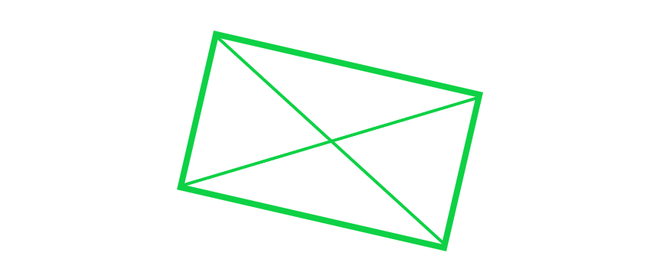For a convex quadrilateral we shall use the formula n(n-3)/2

So, number of diagonals = 4(4-3)/2 = 4/2 = 2

A convex quadrilateral has 2 diagonals.

(ii) A regular hexagon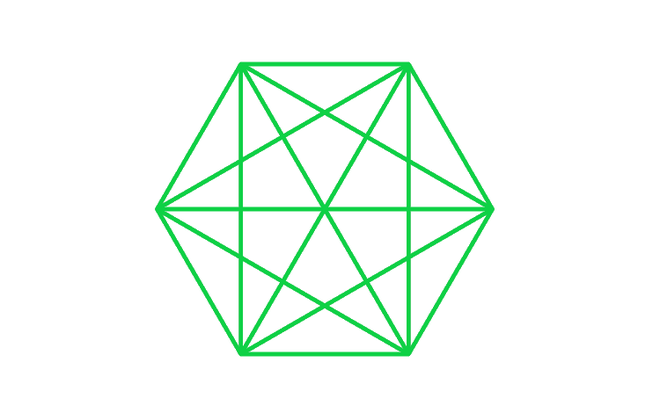For a regular hexagon we shall use the formula n(n-3)/2

So, number of diagonals = 6(6-3)/2 = 18/2 = 9

A regular hexagon has 9 diagonals.

(iii) A triangle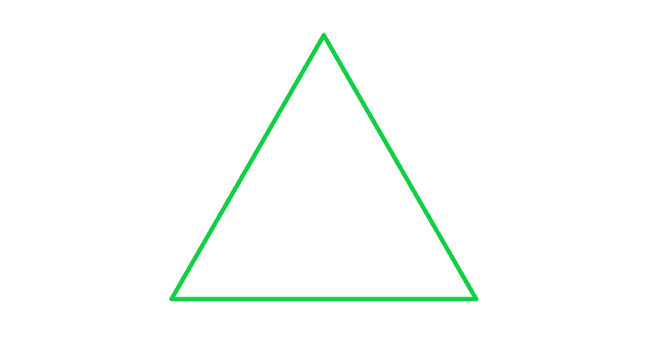For a triangle we shall use the formula n(n – 3)/2

So, number of diagonals = 3(3 -3)/2 = 0/2 = 0

A triangle has no diagonals.

### Question 7. What is a regular polygon? State the name of a regular polygon of

(i) 3 sides

(ii) 4 sides

(iii) 6 sides

Solution:

Regular Polygon: A regular polygon is an enclosed figure. In a regular polygon minimum sides are three.

(i) 3 sides

A regular polygon with 3 sides is known as Equilateral triangle.

(ii) 4 sides

A regular polygon with 4 sides is known as Rhombus.

(iii) 6 sides

A regular polygon with 6 sides is known as Regular hexagon.

My Personal Notes arrow_drop_up
Related Articles